Next: 4.3.5 Mesh Refinement at Up: 4.3 Simulation in FEDOS Previous: 4.3.3 Assembly of the

## 4.3.4 Calculation of the Mechanical Stress

The discretization of the mechanical problem presented in Section 4.2.4 yields the linear system of equations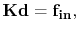(4.96)

with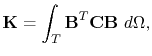(4.97)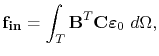(4.98)

Since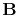,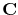, and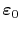are constant within an element, the assembly of (4.96) is performed in FEDOS using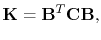(4.99)

and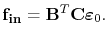(4.100)

The mechanical problem has to be solved each time step after the solution of the vacancy dynamics problem, as shown in Figure 4.3. Thus, for the time step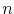, the internal force vector is determined by the electromigration induced strain given in (3.82), so that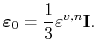(4.101)

From (3.78), the trace of the electromigration strain for each node of the tetrahedron is calculated by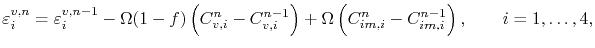(4.102)

resulting in the element strain which is set in (4.101),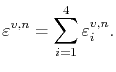(4.103)

The solution of (4.96) yields the interconnect line deformation due to electromigration. Once the displacement field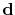is determined, the electromigration induced stress vector for an element is obtained using (4.60),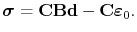(4.104)

The mechanical stress at each node is obtained by an extrapolation from the stress calculated for the elements, given by (4.104). The stress at a particular node is calculated by performing a weighted average of the stress on all elements connected to the node. Considering a node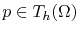and defining the set of all elements which contain the node as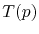, the mechanical stress at the nodeis given by(4.105)

where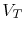is the volume, and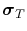is the stress calculated by (4.104) for the element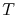.Next: 4.3.5 Mesh Refinement at Up: 4.3 Simulation in FEDOS Previous: 4.3.3 Assembly of the

R. L. de Orio: Electromigration Modeling and Simulation